Rotational Symmetry

Chapter 12 Class 7 Symmetry
Concept wise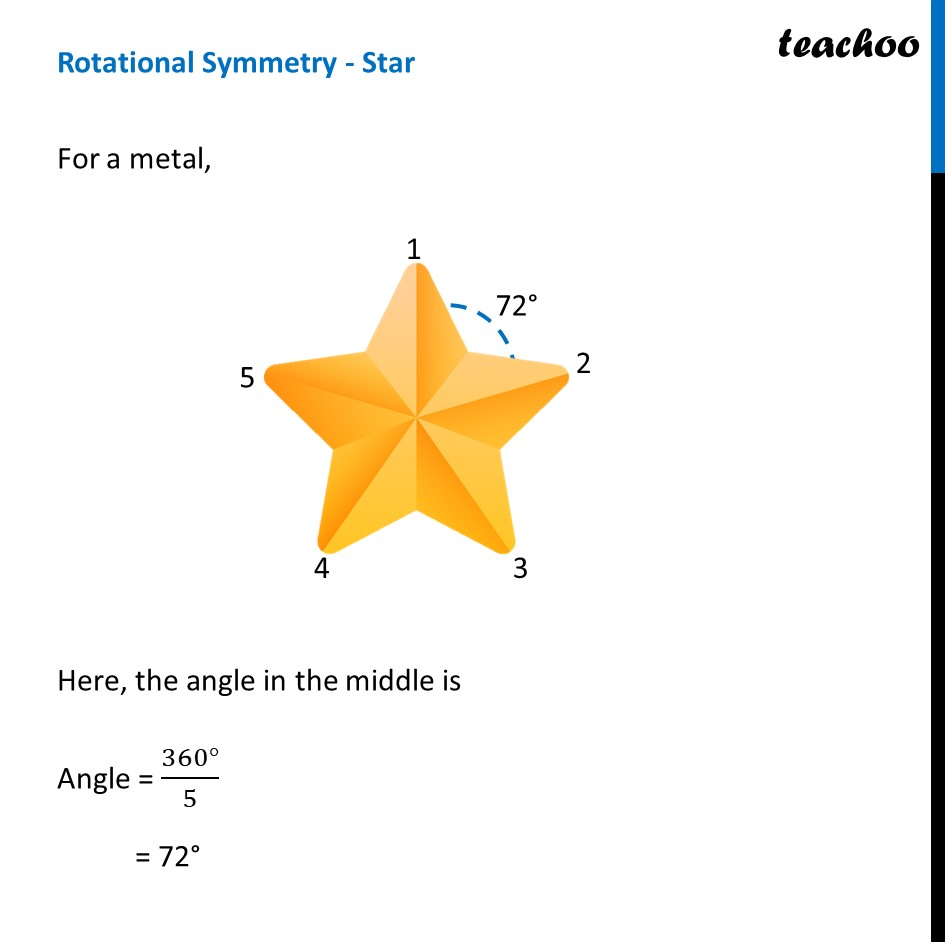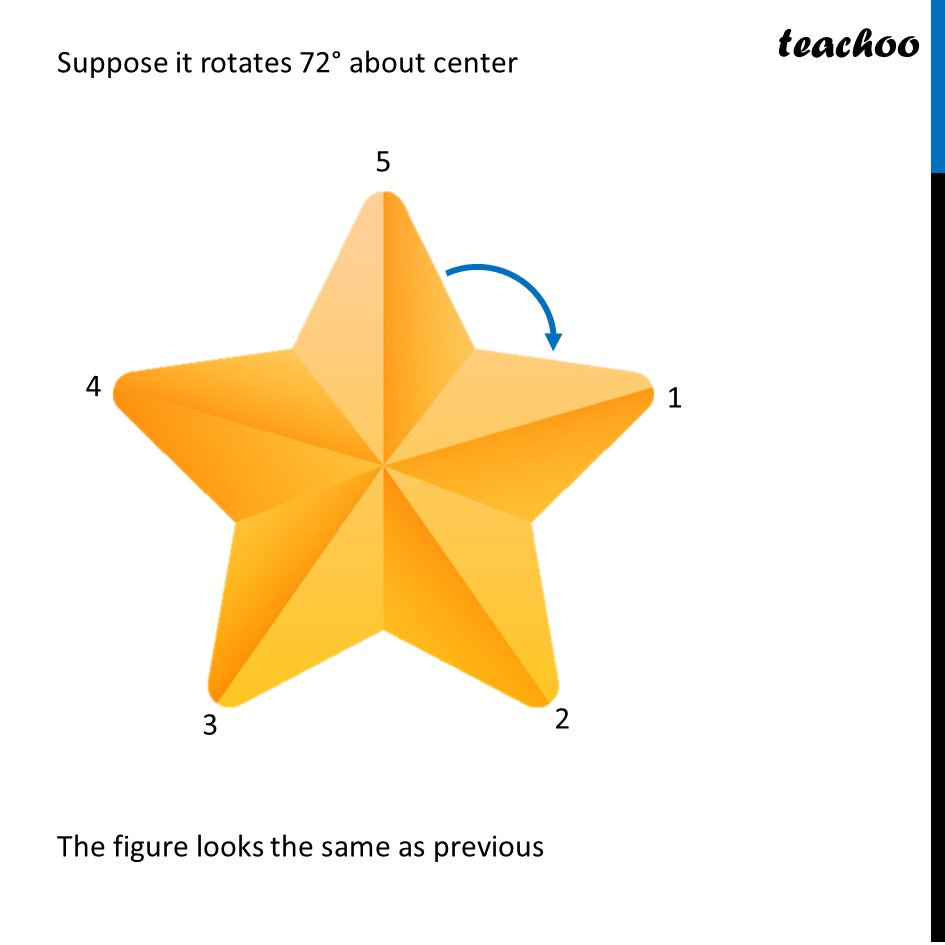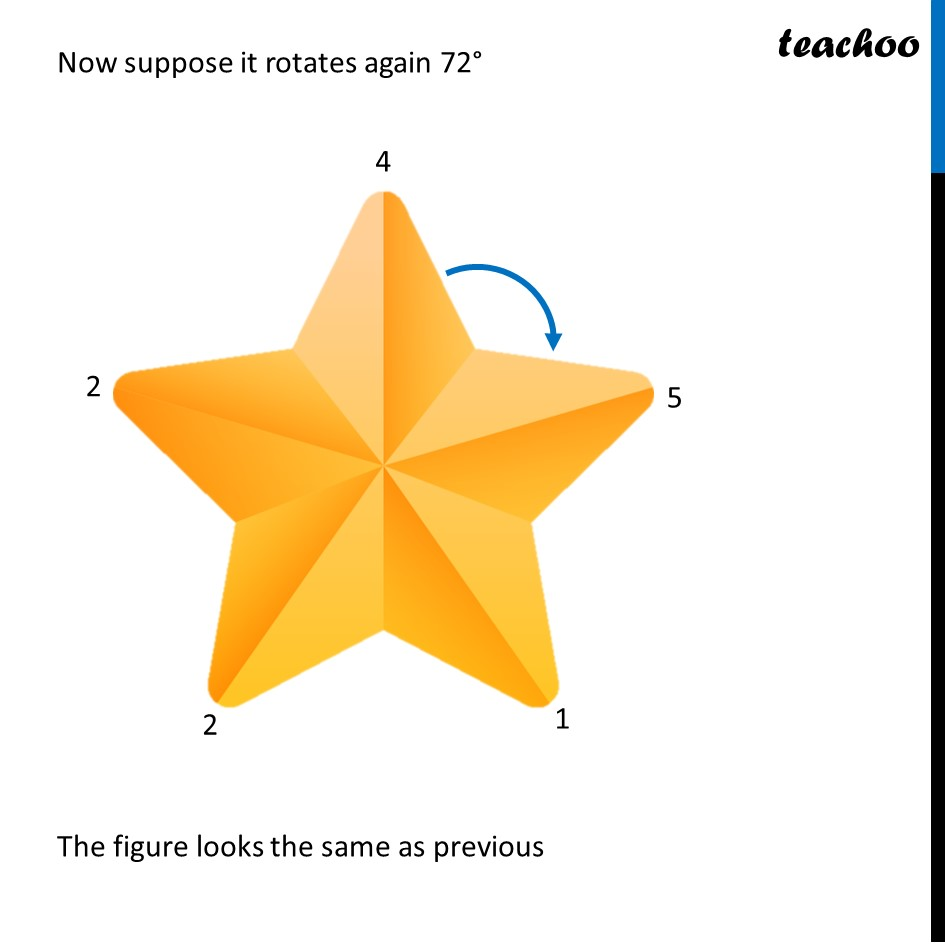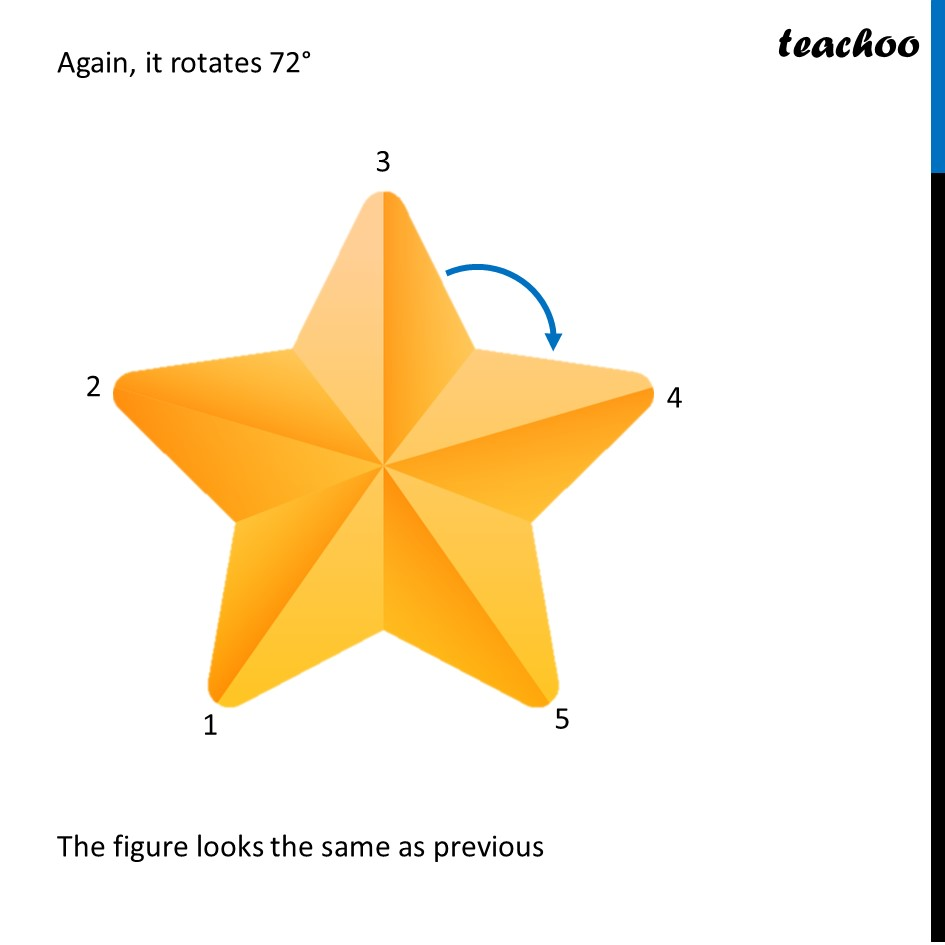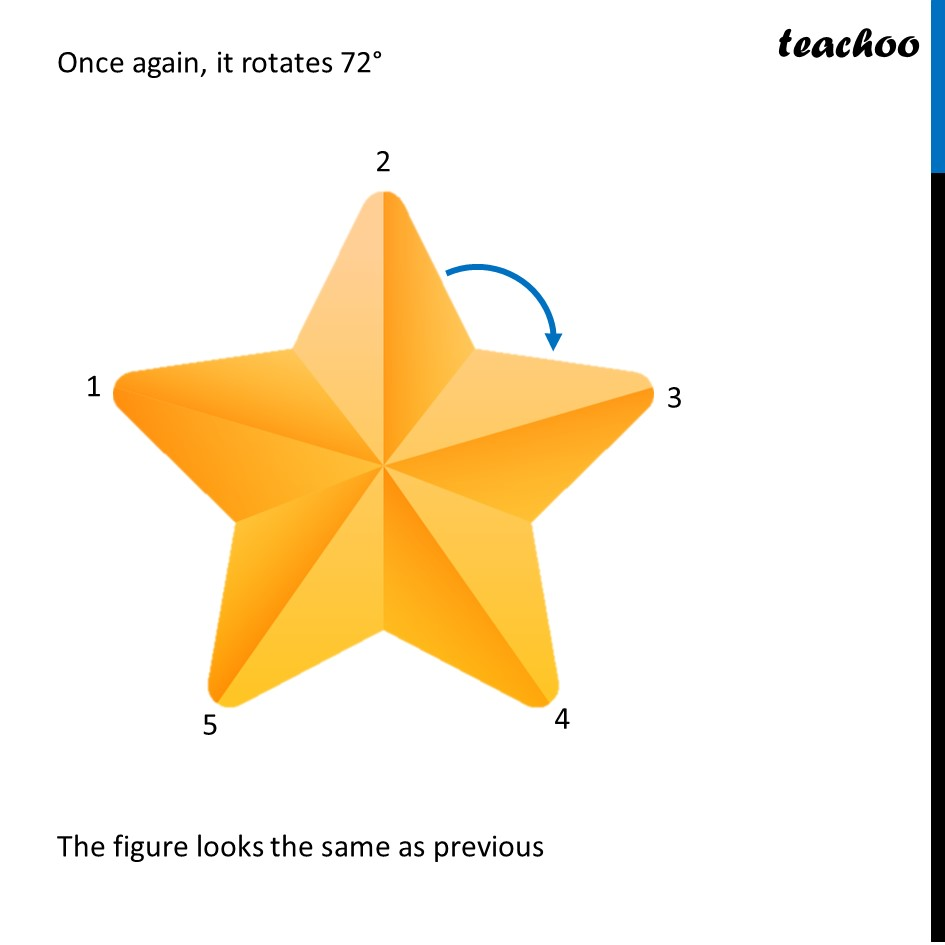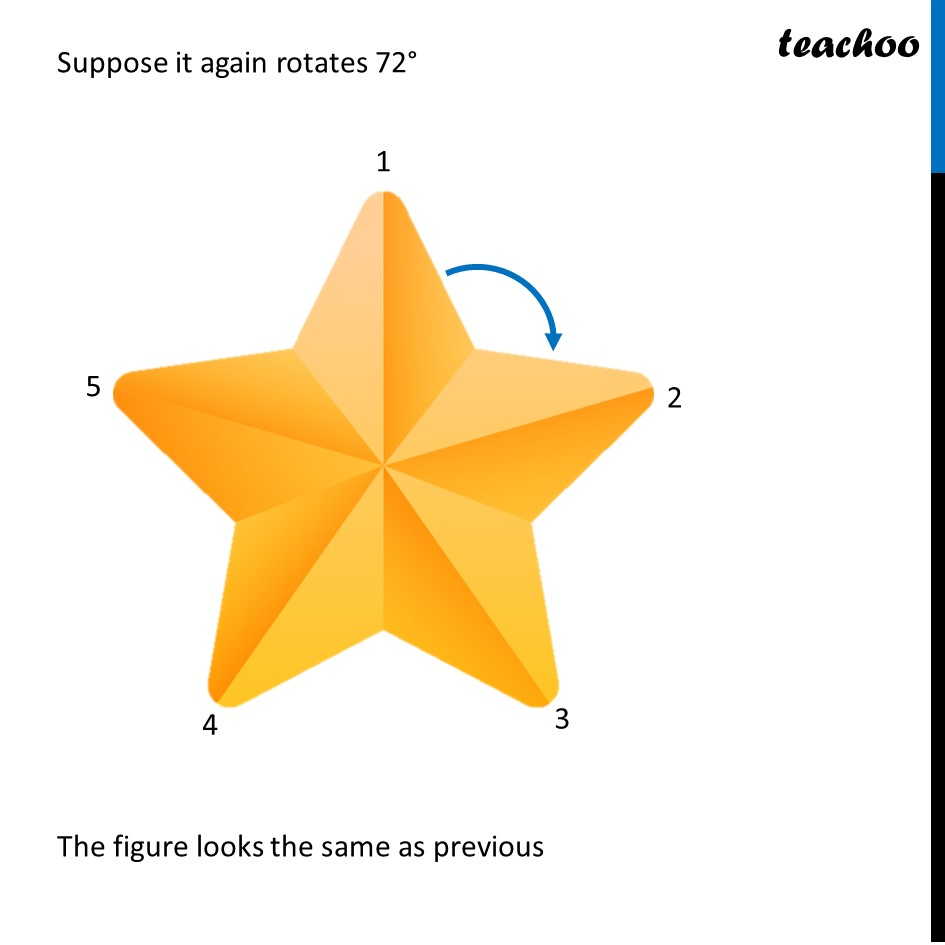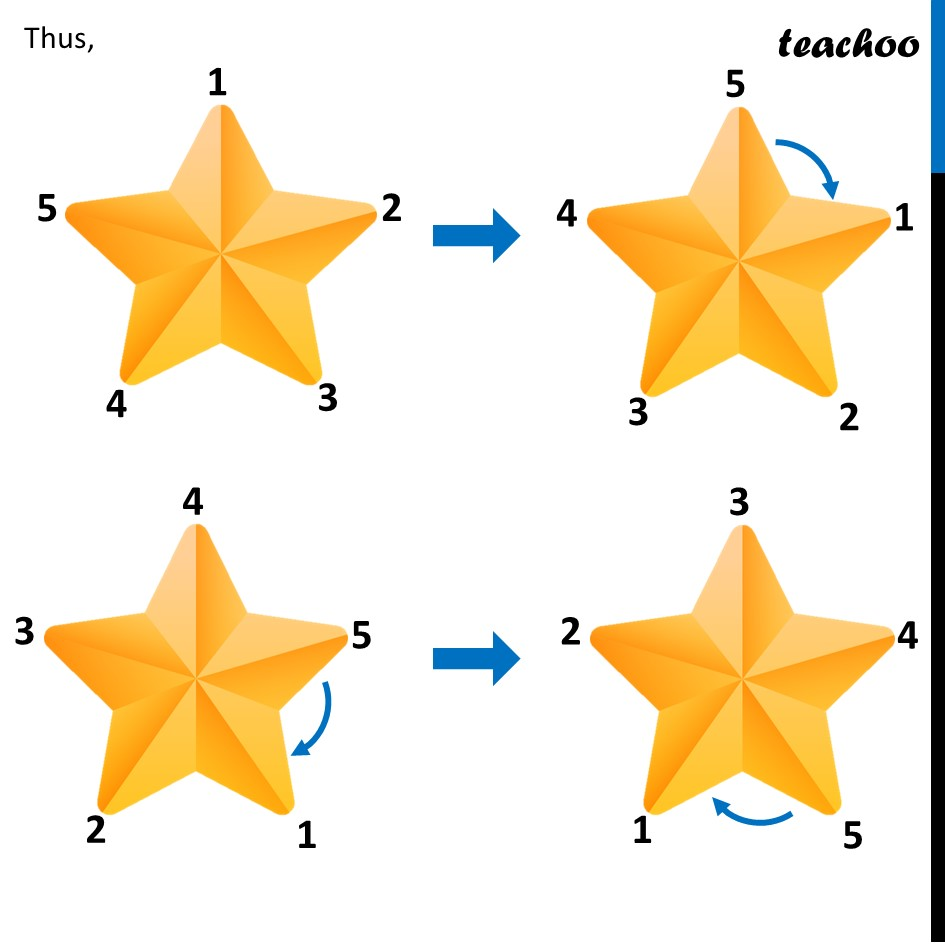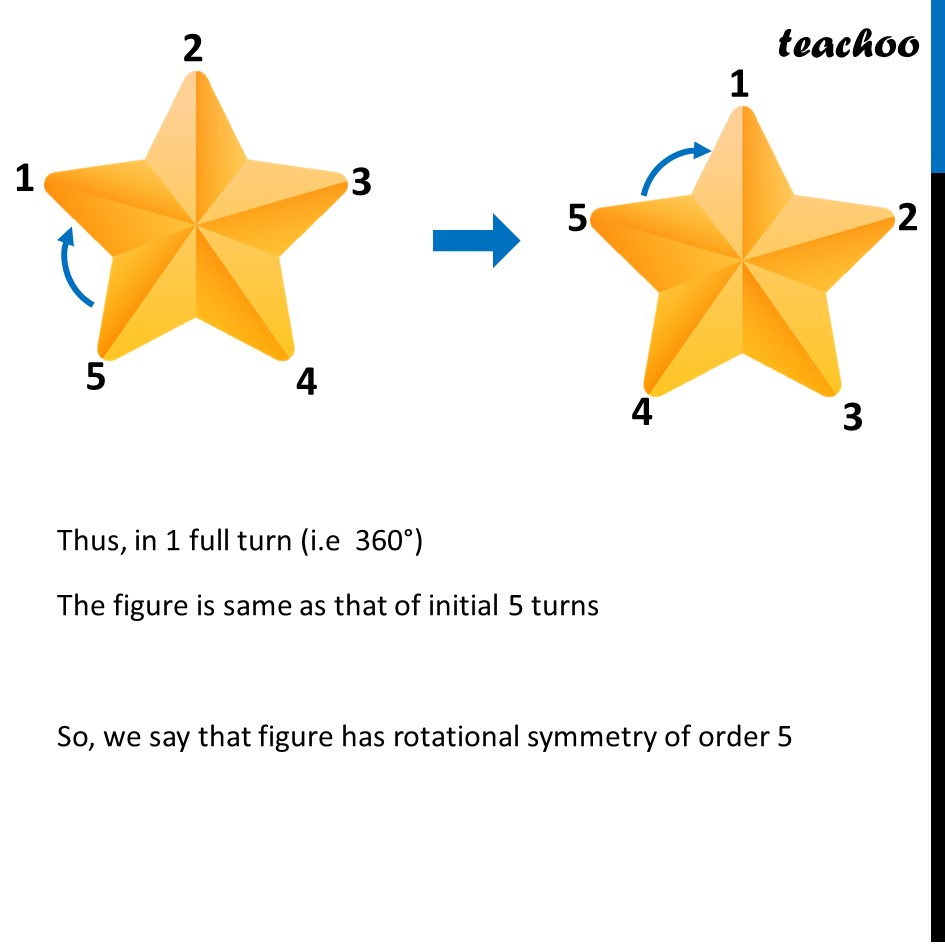Learn in your speed, with individual attention - Teachoo Maths 1-on-1 Class

### Transcript

Rotational Symmetry - StarFor a metal, Here, the angle in the middle is Angle = (360°)/5 = 72° Suppose it rotates 72° about center The figure looks the same as previous Now suppose it rotates again 72° The figure looks the same as previous Again, it rotates 72° The figure looks the same as previous Again, it rotates 72° The figure looks the same as previous Once again, it rotates 72° The figure looks the same as previous Suppose it again rotates 72° The figure looks the same as previous Thus, Thus, in 1 full turn (i.e 360°) The figure is same as that of initial 5 turns So, we say that figure has rotational symmetry of order 5